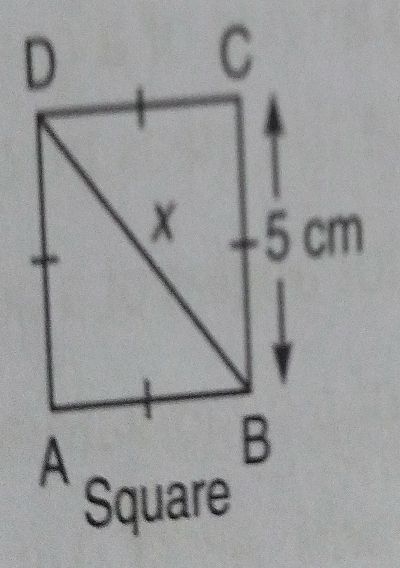"
">

# $ABCD$ is a square, $BC=5\ cm$. Find the value of $x$."

Given: A square $ABCD$ whose side is $5\ cm$.

To do: To find the value of $x$.

Solution:

As given $ABCD$ is a square.

Therefore, $AB=BC=CD=DA=5\ cm$ and diagonal $BD=x$.

On using Pythagoras theorem,

$BD^2=BC^2+CD^2$

$\Rightarrow x^2=BC^2+CD^2$

$\Rightarrow x^2=5^2+5^2$

$\Rightarrow x^2=25+25$

$\Rightarrow x^2=50$

$\Rightarrow x=\sqrt{50}$

$\Rightarrow x=5\sqrt{2}$.

Updated on: 10-Oct-2022

14 Views